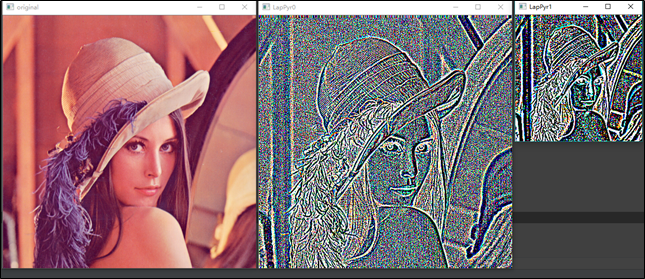## 数字图像处理(21): 图像金字塔(高斯金字塔 与 拉普拉斯金字塔)

1 图像金字塔简介

2 向下取样——pyrDown()

2.1 基础理论

2.2 代码示例

3 向上取样——pyrUp()

3.1 基础理论

3.2 代码示例

4 Laplacian 金字塔

4.1 基础理论

4.2 代码示例

# 1 图像金字塔简介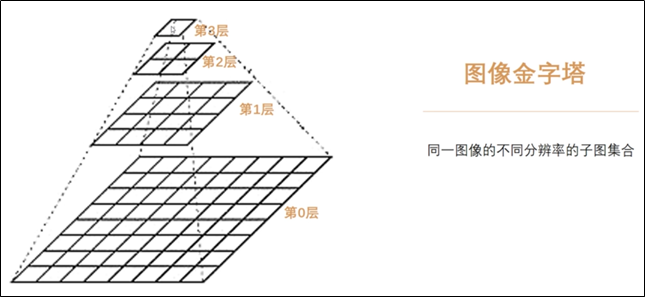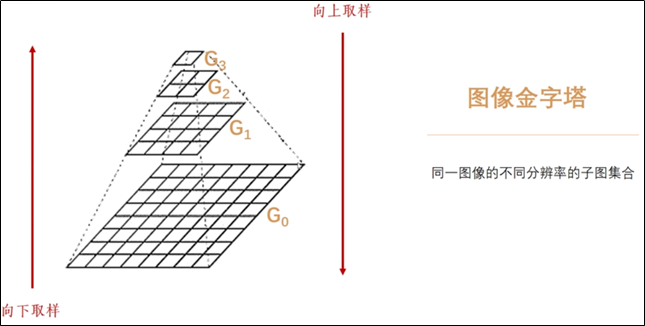# 2 向下取样——pyrDown()

## 2.1 基础理论

（1）对图像Gi进行高斯卷积核（高斯滤波）；

（2）删除所有的偶数行和列。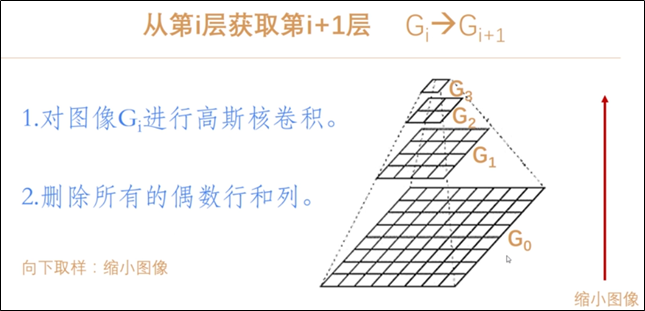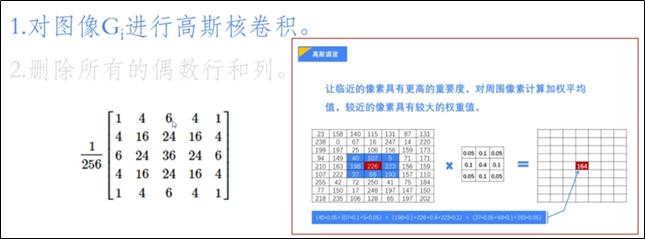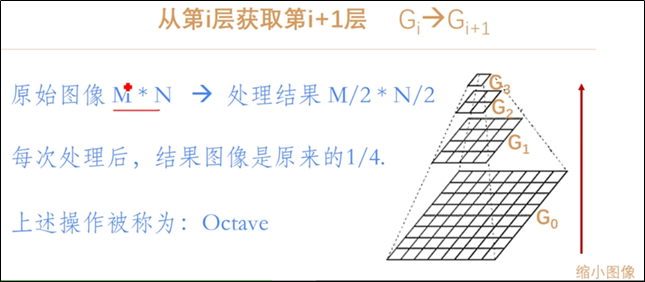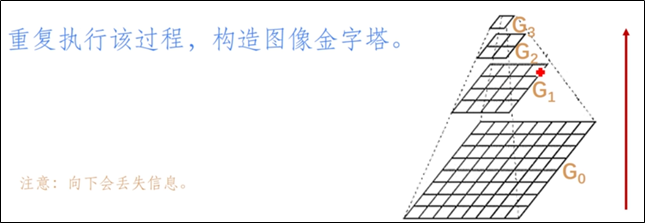：由于每次向下取样会删除偶数行和列，所以它会不停地丢失图像的信息。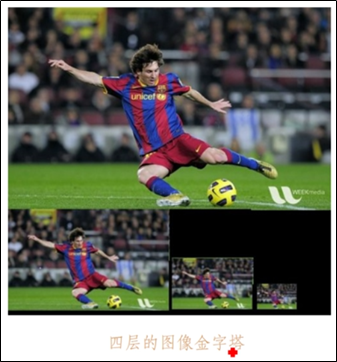## 2.2 代码示例

dst = pyrDown(src[, dst[, dstsize[, borderType]]])

src 表示输入图像；

dst 表示输出图像，和输入图像具有一样的尺寸和类型；

dstsize 表示输出图像的大小，默认值为Size()；

borderType 表示像素外推方法，详见cv::bordertypes 。

（1）一次向下采样

# -*- coding: utf-8 -*-
import cv2
import numpy as np
import matplotlib.pyplot as plt

#读取原始图像

#图像向下取样
r = cv2.pyrDown(img)

#显示图像
cv2.imshow('original', img)
cv2.imshow('PyrDown', r)

cv2.waitKey()
cv2.destroyAllWindows()（2）多次向下采样

# -*- coding: utf-8 -*-
import cv2
import numpy as np
import matplotlib.pyplot as plt

#读取原始图像

#图像向下取样
r1 = cv2.pyrDown(img)
r2 = cv2.pyrDown(r1)
r3 = cv2.pyrDown(r2)

#显示图像
cv2.imshow('original', img)
cv2.imshow('PyrDown1', r1)
cv2.imshow('PyrDown2', r2)
cv2.imshow('PyrDown3', r3)

cv2.waitKey()
cv2.destroyAllWindows()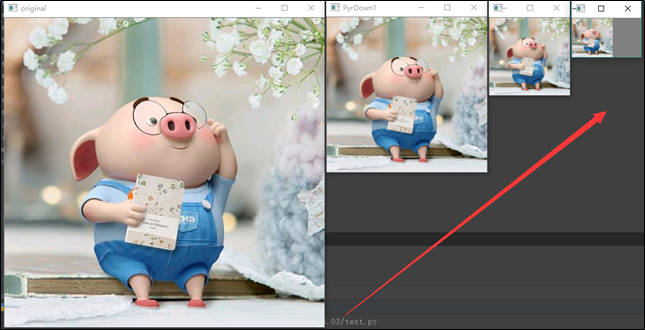# 3 向上取样——pyrUp()

## 3.1 基础理论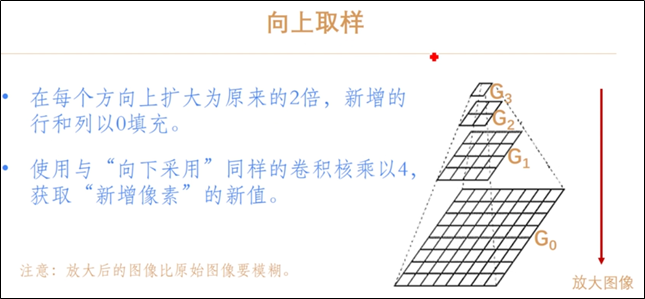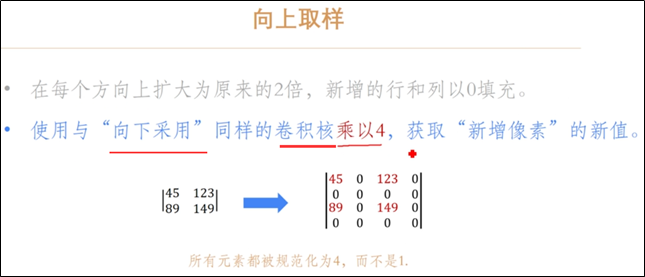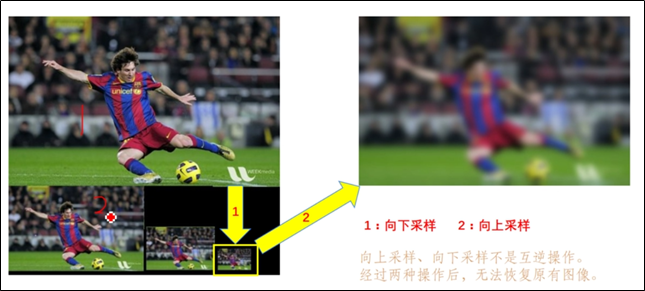## 3.2 代码示例

dst = pyrUp(src[, dst[, dstsize[, borderType]]])

src 表示输入图像；

dst 表示输出图像，和输入图像具有一样的尺寸和类型；

dstsize 表示输出图像的大小，默认值为Size()；

borderType 表示像素外推方法，详见cv::bordertypes 。

# -*- coding: utf-8 -*-
import cv2
import numpy as np
import matplotlib.pyplot as plt

#读取原始图像

#图像向上取样
r = cv2.pyrUp(img)

#显示图像
cv2.imshow('original', img)
cv2.imshow('PyrUp', r)
cv2.waitKey()
cv2.destroyAllWindows()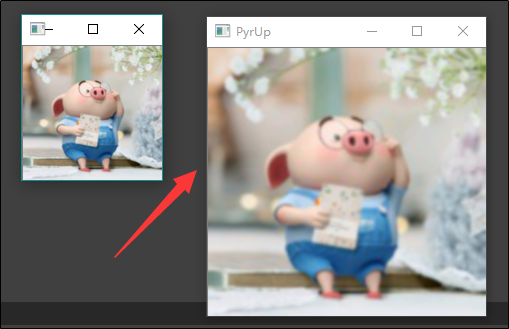# 4 Laplacian 金字塔

## 4.1 基础理论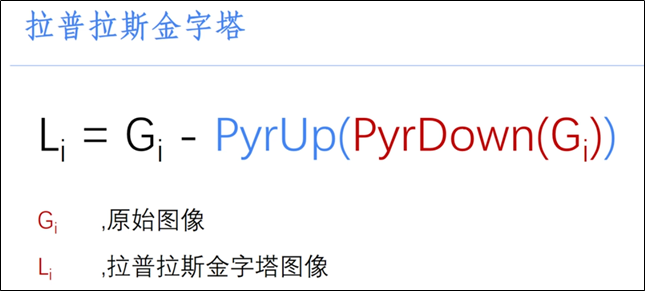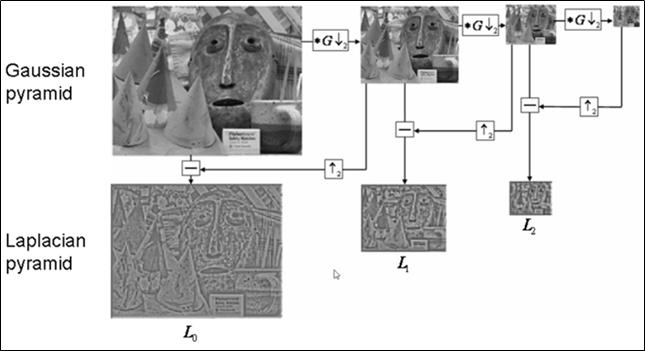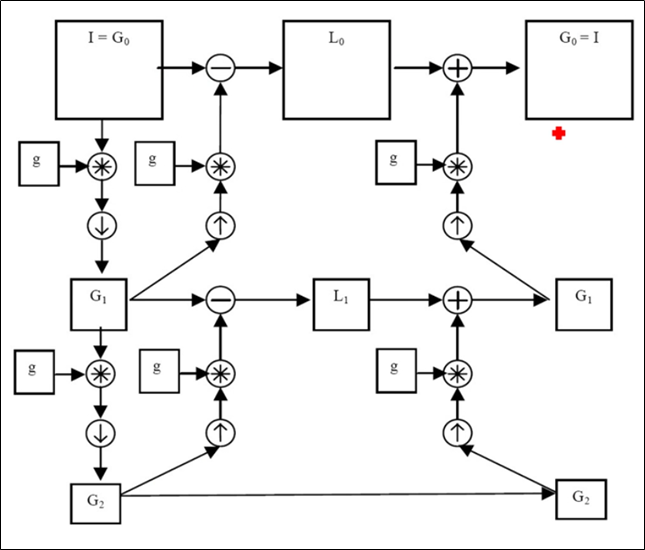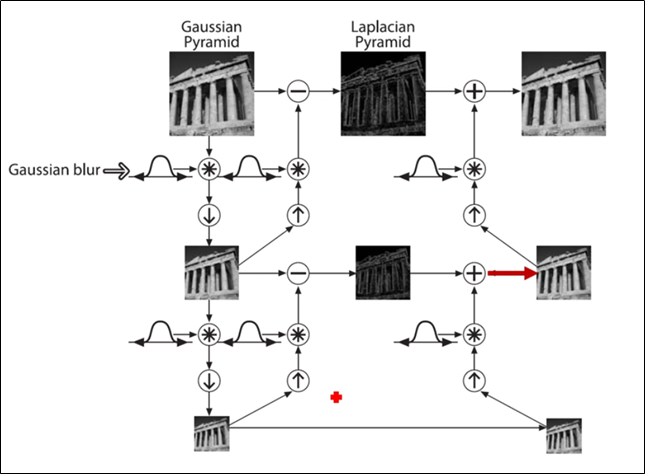## 4.2 代码示例

（1） 拉普拉斯第0层

# -*- coding: utf-8 -*-
import cv2
import numpy as np
import matplotlib.pyplot as plt

#读取原始图像

#图像向下取样
r1 = cv2.pyrDown(img)

#图像向上取样
r2 = cv2.pyrUp(r1)

# 拉普拉斯第0层
LapPyr0 =img-r2

#显示图像
cv2.imshow('original', img)
cv2.imshow('LapPyr', LapPyr0)
cv2.waitKey()
cv2.destroyAllWindows()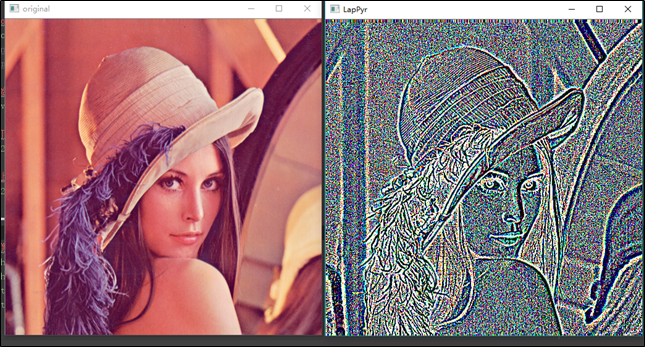（2） 拉普拉斯第0层和拉普拉斯第1层

# -*- coding: utf-8 -*-
import cv2
import numpy as np
import matplotlib.pyplot as plt

#读取原始图像

#图像向下取样
r1 = cv2.pyrDown(img)

#图像向上取样
r2 = cv2.pyrUp(r1)

# 拉普拉斯第0层
LapPyr0 =img-r2

#图像向下取样
r3 = cv2.pyrDown(r1)

#图像向上取样
r4 = cv2.pyrUp(r3)

# 拉普拉斯第1层
LapPyr1 =r1-r4

#显示图像
cv2.imshow('original', img)
cv2.imshow('LapPyr0', LapPyr0)
cv2.imshow('LapPyr1', LapPyr1)
cv2.waitKey()
cv2.destroyAllWindows()